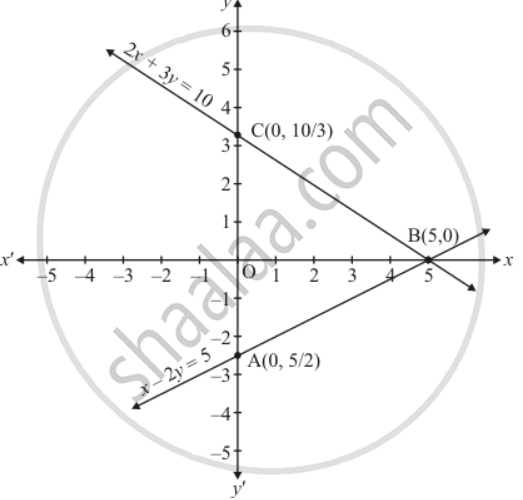Share

# Solve the Following Systems of Equations Graphically: X − 2y = 5 2x + 3y = 10 - CBSE Class 10 - Mathematics

ConceptGraphical Method of Solution of a Pair of Linear Equations

#### Question

Solve the following systems of equations graphically:

x − 2y = 5

2x + 3y = 10

#### Solution

The given equations are

x - 2y = 5  ...(1)

2x + 3y = 10 ...(2)

Putting x = 0 in equation (1) we get

=>  0 - 2y = 5

=> y = -5/2

x = 0, y = -5/2

Putting y = 0 in equation (1) we get

=> x + 2 xx 0 = 5

=> x = 5

x = 5, y = 0

Use the following table to draw the graph.

 x 0 5 y -5/2 0

Draw the graph by plotting the two points A(0, -5/2) and B(5,0) from tableGraph the equation (2)

=>2x + 3y = 10  ...(2)

Putting x = 0 i equation (ii) we get

=> 2 xx 0 + 3y =10

=> y = 10/3

x = 0, y = 10/3

Putting y = 0 in equation (2) we get

=>2x + 3 xx 0 = 10

=>  x = 5

x = 5, y = 0

Use the following table to draw the graph.

 x 0 5 y 10/3 0

Draw the graph by plotting the two points C(0,10/3) and B(5,0) from table

The two lines intersects at point B(5,0)

Hence x = 5, y = 0 is the solution

Is there an error in this question or solution?

#### APPEARS IN

Solution Solve the Following Systems of Equations Graphically: X − 2y = 5 2x + 3y = 10 Concept: Graphical Method of Solution of a Pair of Linear Equations.
S